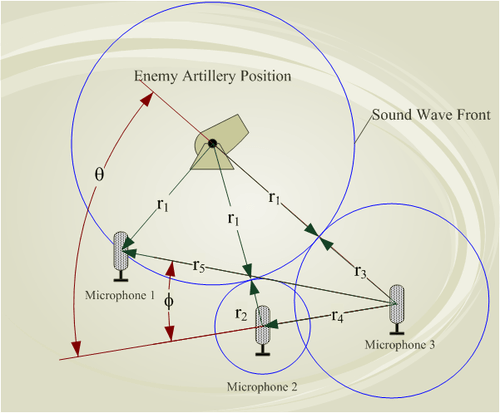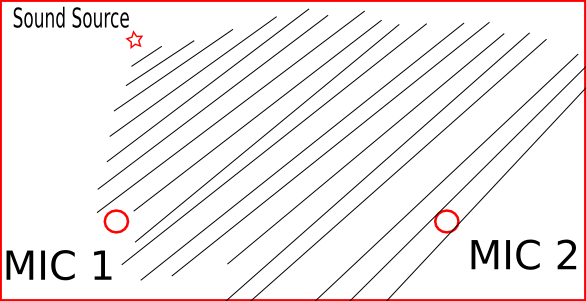# Sound triangulation using cheap microphones

Sound triangulation is a method of determining where a sound originated from. Triangulation can give the location of a sound source in 2-d space. A sound wave travels out from its point of origin as a sphere. Trilateration is another method a locating the source of a sound. It is modeled around the fact that sound waves travel out spherically. See the below images for a better description of what I mean.Trilateration

Picture source : http://en.wikipedia.org/wiki/Trilateration

It had very useful military applications:artillery

Picture source : http://en.wikipedia.org/wiki/Sound_ranging

The equations for the Trilateration model of sound location were a bit difficult for me to understand well enough to build a project around them. Triangulation solves this problem by using a much simpler model of sound location. It assumes that all sound waves from a sound source travel out in a planer way(straight lines) instead of in a spherical way. This greatly simplifies the equation to a few variables that are related by basic trigonometry.planer sound diagram

The equation is simplified to :$\Delta t = \frac{x sin{\theta}}{c}$

where:$\Delta t$ is the time difference in seconds$x$ is the distance between the microphones in meters$\theta$ is the angle between the baseline of the microphones and the incident sound, in degrees$c$ is the speed of sound

This blog is incomplete, will add in project details later.

This entry was posted in Ideas, Uncategorized. Bookmark the permalink.